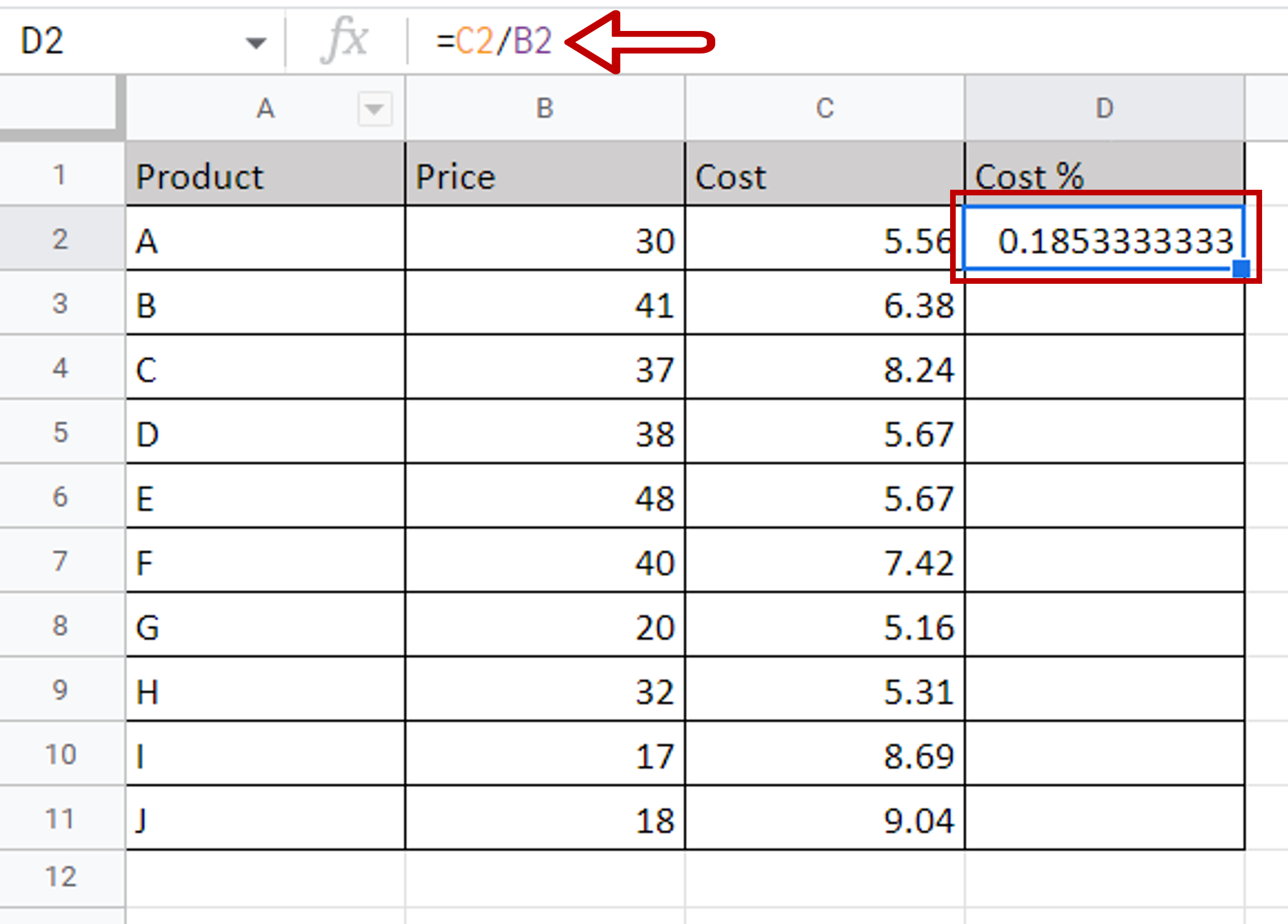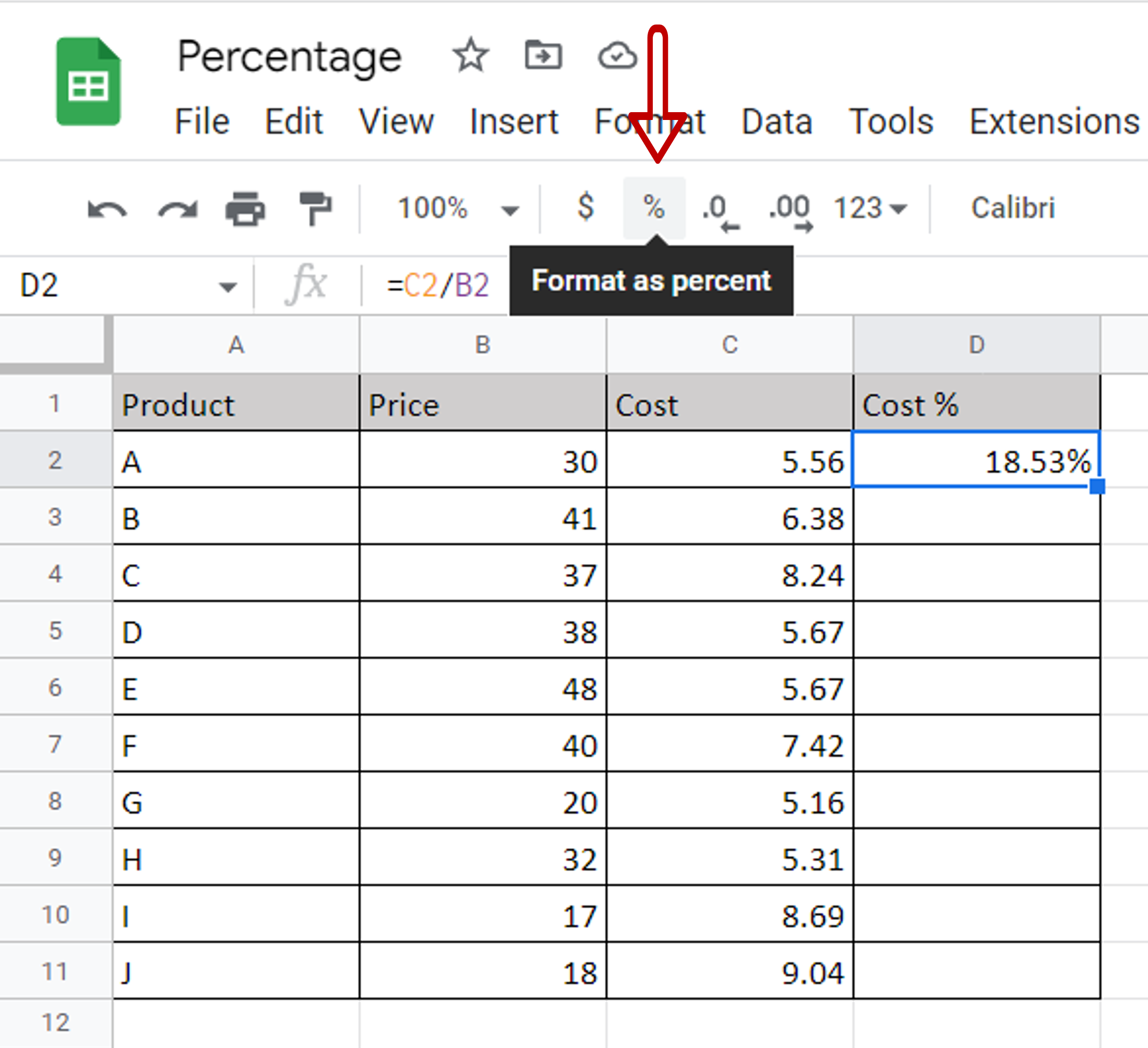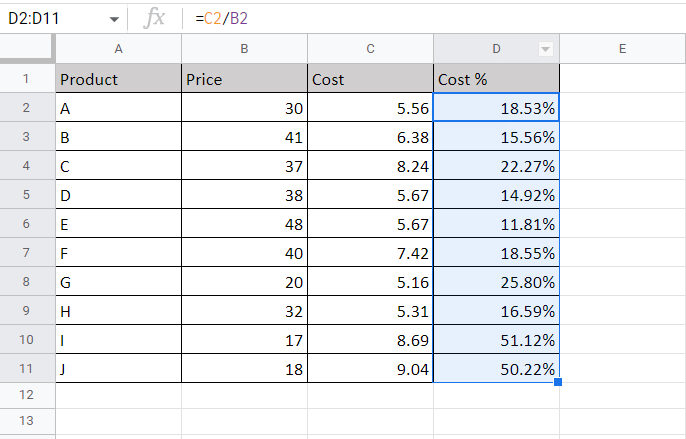# How to calculate percentage in Google Sheets

You can watch a video tutorial here.Google Sheets is frequently used for calculations and supports all basic mathematical operations.  Here we will see how to find the percentage of two columns of numbers.

### Step 1 – Find the ratio of the numbers– In the destination cell type the formula using cell references:

=Cost/Price

### Step 2 – Format the number as a percentage– Select the cell with the value
– Click on the percentage sign (%) on the toolbar
OR
Go to Format> Number>Percent

### Step 3 – Copy the formula– Using the fill handle from the first cell, drag the formula to the remaining cells
OR
a) Select the cell with the formula and press Ctrl+C or choose Copy from the context menu (right-click)
b) Select the rest of the cells in the column and press Ctrl+V or choose Paste from the context menu (right-click)